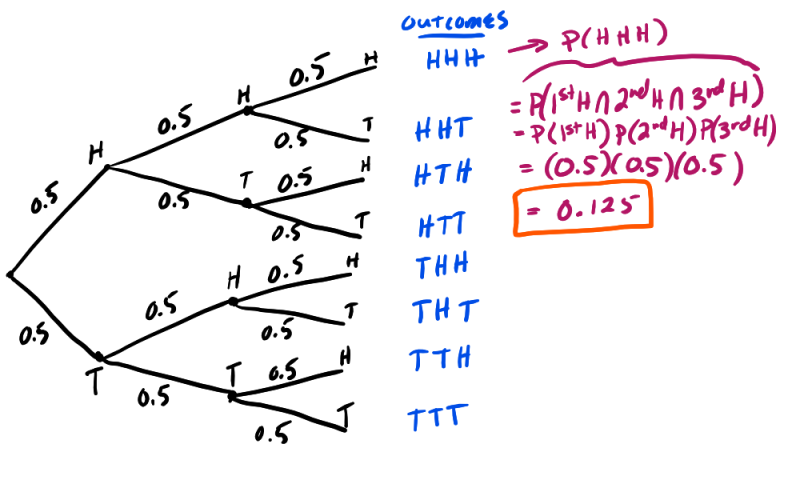Multiplication Rule & Tree Diagrams

Multiplication Rule for Independent Events

If knowing that event A occurs does not change the probability that event B occurs or vice versa, then we can say that events A and B are independent. If two events are independent, then one can calculate the probability that both events occur by using the following formula:

If A and B are independent events: P(A and B)  = P(A)P(B)

This rule can be expanded beyond just two events. For example, if A, B, and C are all independent events, then the following formula holds true:

If A, B, and C are independent events: P(A B  C)  = P(A)P(B)P(C)

Tree Diagrams

Tree diagrams are a powerful tool that allows one to visualize all the possible outcomes of a random activity or experiment. Each branch in a tree diagram represents a possible outcome. Furthermore, once the tree diagram is drawn and outcomes have been established, tree diagrams help one calculate the corresponding probabilities of each outcome.EXAMPLES

In rolling a fair die, what is the chance of rolling an even number or a number less than 3?

Solution:

A = Getting a {2 or lower} number = {1,2}

P(A) = 2/6

B= Getting an even number = {2,4,6}

P(B) = 3/6

A ∩ B = {2}

P(A ∩ B) = 1/6

Therefore,   P(A ∪ B) = P(A) + P(B) − P(A ∩ B)  = 2/6 + 3/6 - 1/6 = 4/6 = 2/3

2. In Chesterfield High School, the probability a randomly chosen student is a freshman is 0.29, and the probability a randomly chosen student is a senior is 0.21. Calculate the probability that a randomly chosen student at Chesterfield High school is either a sophomore or a senior.
P(freshman or senior) = P(freshman) + P(senior) - P(freshman and senior)
However, because a student can not be both a freshman and senior, these two events are disjoint. Therefore, P(freshman and senior) = 0. Simplifying:
P(freshman or senior) = P(freshman) + P(senior) = 0.29 + 0.21 = 0.50

3. John has an extra ticket to tonight's concert. He randomly texts two friends, Jane and Molly. Neither knows each other. He estimates the probability that Jane will say yes to buying the ticket and 0.4 and Molly will say yes with a probability of 0.1.
a. John is nervous that both will respond back and say they want to go. Using John's estimates, determine the probability that this will happen?
P(J and M) = P(J)P(M) = (0.4)(0.1) = 0.04
b. Calculate the probability that either Jane or Molly will go.
P(J or M) = P(J) + P(M) - P(J and M) = 0.4 + 0.1 - 0.04 = 0.46
c. Calculate the probability that neither Jane or Molly decide to go?
P(Jc and Mc) = P(Jc)P(Mc ) = (0.6)(0.9) = 0.54

3. Katie is running very late to work. She believes that the only way she will get to work on time is if the three lights she hits on the way to work are all green. The probability that any one light is green is 0.6. This probability is the same for all three lights. Calculate the probability that she hits all green lights on the way to work?
P(1st Green and 2nd Green and 3rd Green) = P(1st Green)P(2nd Green)P(3rd Green) = (0.6)(0.6)(0.6) = 0.63 = 0.216